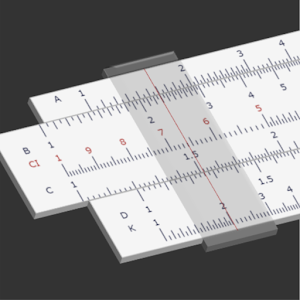\$0.99

# Android Giveaway of the Day - Smart Slide Rule

An app simulating a slide rule!
\$0.99 期限切れ
ユーザーの評価: 4 (100%) 0 (0%)

このgiveawayオファーはすでに期限が切れています。Smart Slide Ruleが今紹介されています。

* An app simulating a slide rule! *

Smart Slide Rule reproduces a slide rule (slipstick) with A, B, C, CI, D, S, L, T, and K scales.
A slide rule is a mechanical analog calculating tool that uses the principle of logarithms.
The slide rule appears as an important prop in the movies "Apollo 13" and "The Wind Rises".
This application reproduces a slide rule on the screen of your device.
The front side of the slide strip corresponds to the B, C, and CI scales, and the back side corresponds to the S, L, and T scales.
You can move the slide strip and the cursor.

* With instructions on how to use the slide rule! *

The slide rule uses a logarithmic scale to calculate multiplication, division, exponentiation, square root, and trigonometric and logarithmic functions.
This application features an explanation of how to use the slide rule, so you can learn how to calculate.
Since the calculation is performed in pixels of the device screen, the accuracy of the calculation cannot be guaranteed.

TAPO

Tools

2.0.0

3.6M

Everyone

5.1 and up

## コメント Smart Slide Rule

Thank you for voting!